Ex 6.1

Chapter 6 Class 12 Application of Derivatives
Serial order wise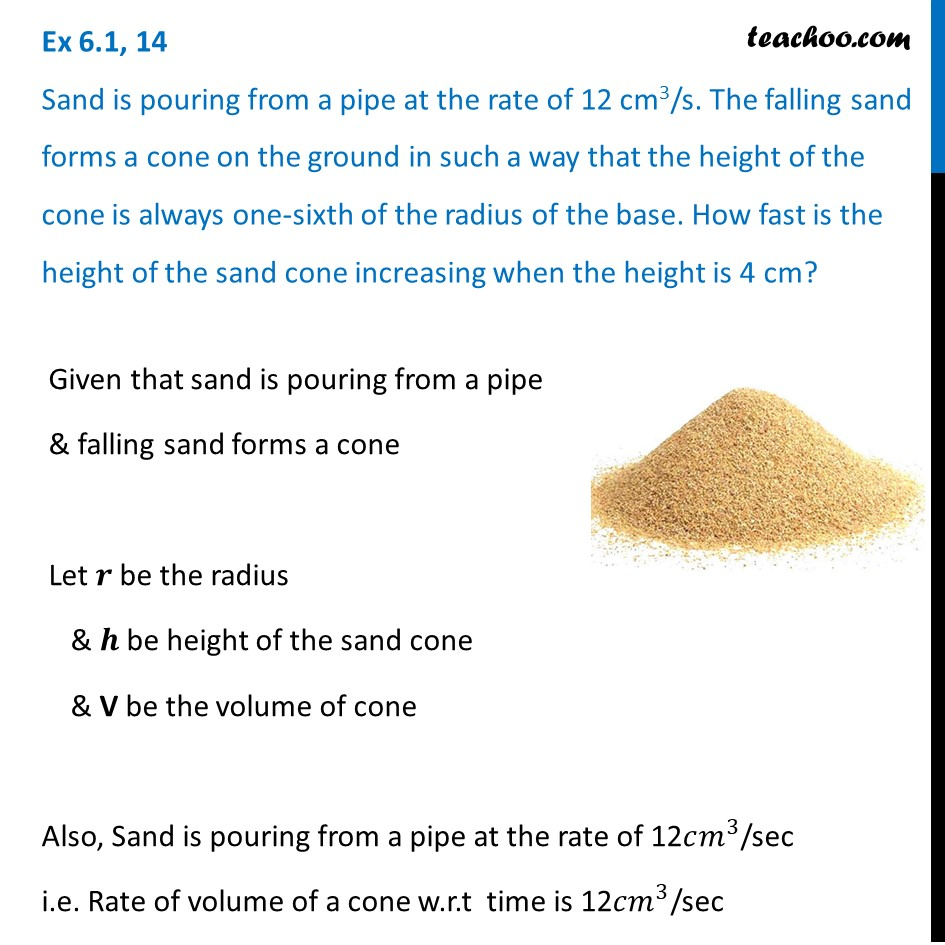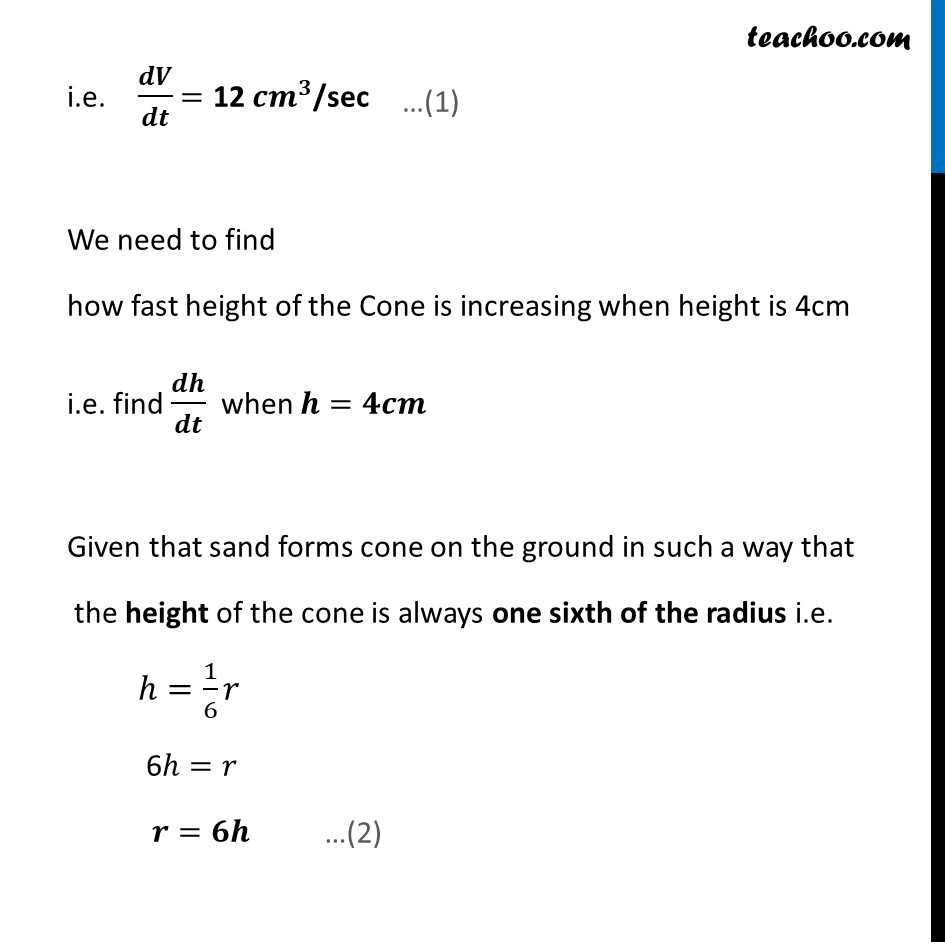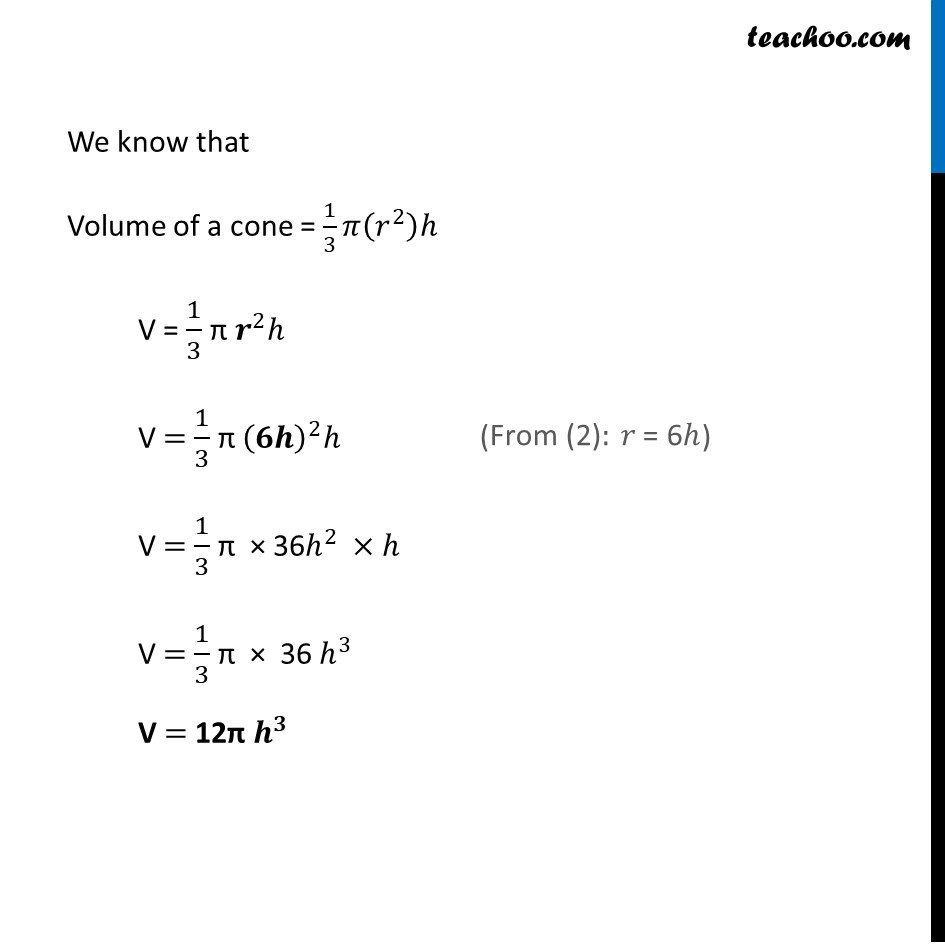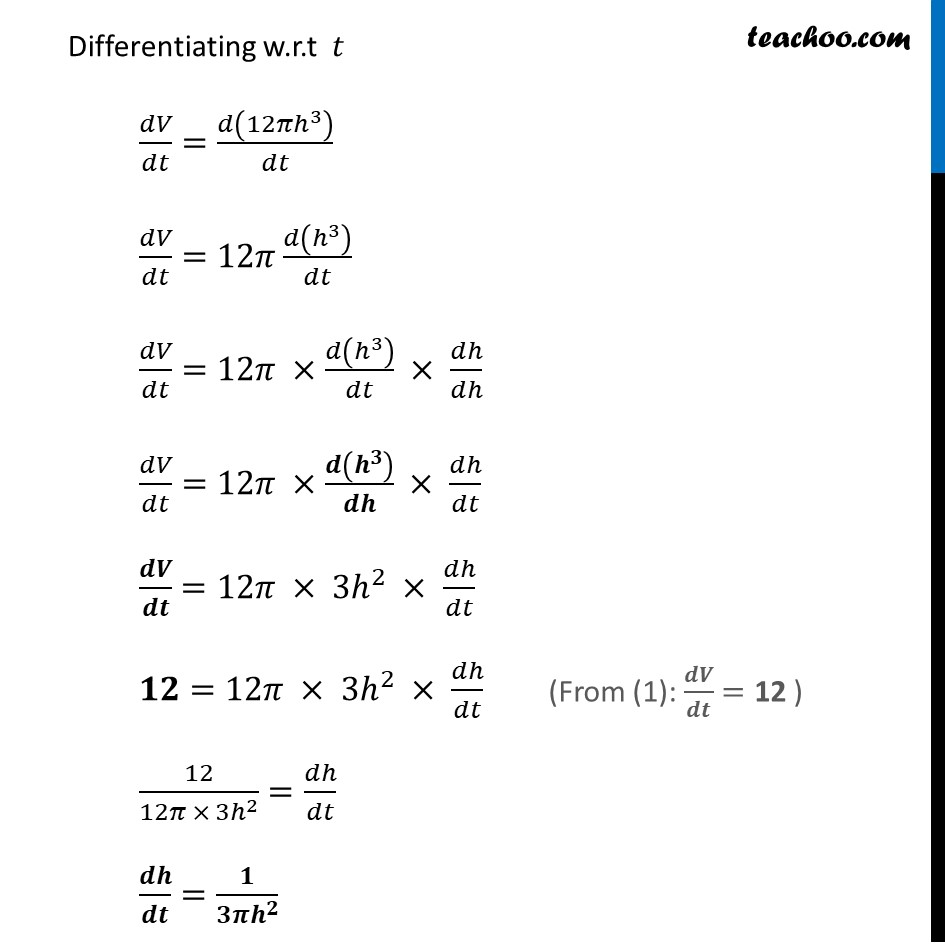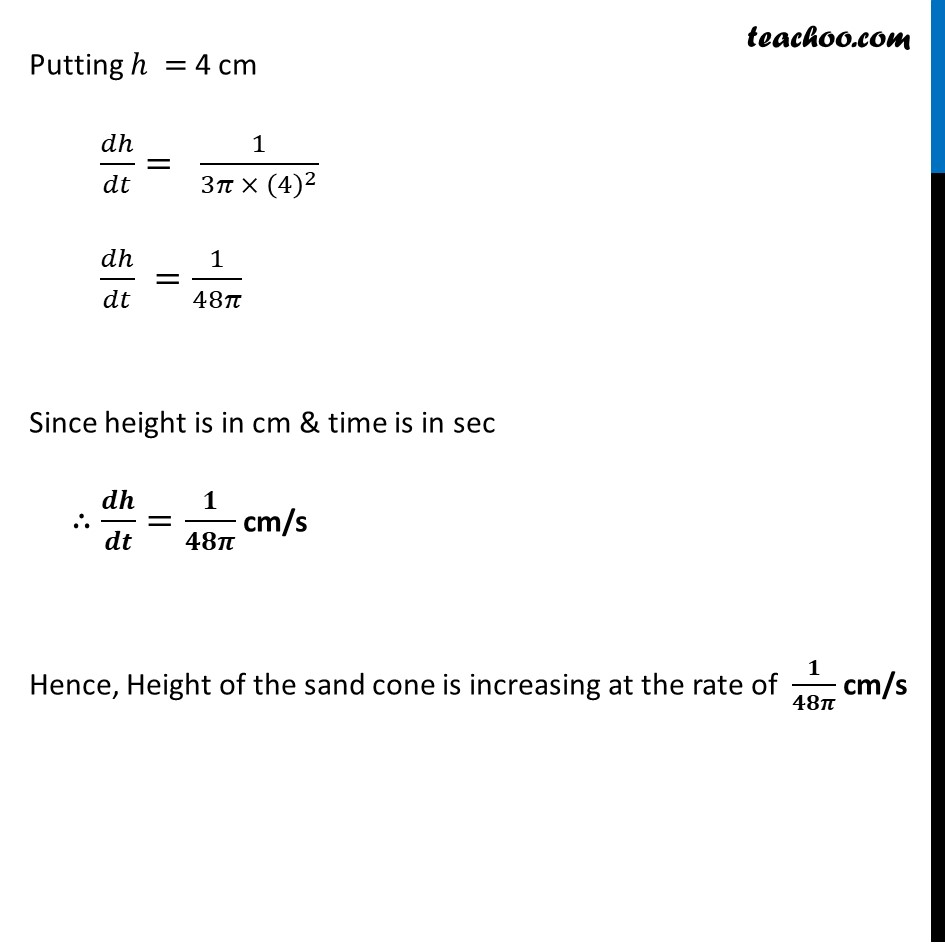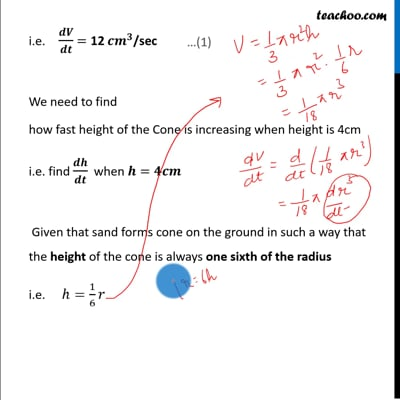This video is only available for Teachoo black users

Solve all your doubts with Teachoo Black (new monthly pack available now!)

### Transcript

Ex 6.1, 14 Sand is pouring from a pipe at the rate of 12 cm3/s. The falling sand forms a cone on the ground in such a way that the height of the cone is always one-sixth of the radius of the base. How fast is the height of the sand cone increasing when the height is 4 cm? Given that sand is pouring from a pipe & falling sand forms a cone Let 𝒓 be the radius & 𝒉 be height of the sand cone & V be the volume of cone Also, Sand is pouring from a pipe at the rate of 12𝑐𝑚^3/sec i.e. Rate of volume of a cone w.r.t time is 12𝑐𝑚^3/sec i.e. 𝒅𝑽/𝒅𝒕 ="12" 𝒄𝒎^𝟑 "/sec" We need to find how fast height of the Cone is increasing when height is 4cm i.e. find 𝒅𝒉/𝒅𝒕 when 𝒉=𝟒𝒄𝒎 Given that sand forms cone on the ground in such a way that the height of the cone is always one sixth of the radius i.e. ℎ=1/6 𝑟 6ℎ=𝑟 𝒓=𝟔𝒉 We know that Volume of a cone = 1/3 𝜋(𝑟^2 )ℎ V = 1/3 π〖 𝒓〗^2 ℎ V = 1/3 π (𝟔𝒉)^2 ℎ V = 1/3 π × 36ℎ^2 ×ℎ V = 1/3 π × 36 ℎ^3 V = 12π 𝒉^𝟑 (█("From (2)" : 𝑟" = 6" ℎ)) Differentiating w.r.t 𝑡 𝑑𝑉/𝑑𝑡=𝑑(12𝜋ℎ^3 )/𝑑𝑡 𝑑𝑉/𝑑𝑡=12𝜋 𝑑(ℎ^3 )/𝑑𝑡 𝑑𝑉/𝑑𝑡=12𝜋 ×𝑑(ℎ^3 )/𝑑𝑡 × 𝑑ℎ/𝑑ℎ 𝑑𝑉/𝑑𝑡=12𝜋 ×𝒅(𝒉^𝟑 )/𝒅𝒉 × 𝑑ℎ/𝑑𝑡 𝒅𝑽/𝒅𝒕=12𝜋 × 3ℎ^2 × 𝑑ℎ/𝑑𝑡 𝟏𝟐=12𝜋 × 3ℎ^2 × 𝑑ℎ/𝑑𝑡 12/(12𝜋 × 3ℎ^2 )=𝑑ℎ/𝑑𝑡 𝒅𝒉/𝒅𝒕=𝟏/(𝟑𝝅𝒉^𝟐 ) (From (1): 𝒅𝑽/𝒅𝒕 ="12" ) Putting ℎ = 4 cm 𝑑ℎ/𝑑𝑡= 1/(3𝜋 × (4)^2 ) 𝑑ℎ/𝑑𝑡 =1/48𝜋 Since height is in cm & time is in sec ∴ 𝒅𝒉/𝒅𝒕=𝟏/𝟒𝟖𝝅 cm/s Hence, Height of the sand cone is increasing at the rate of 𝟏/𝟒𝟖𝝅 cm/s